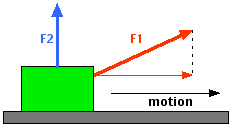# Circular Motion of a ball

http://img149.imageshack.us/img149/7007/untitledpq6.png [Broken]

A ball is spun at a constant velocity.

a. How much work is done by the cord in one revolution?

b. The speed of the ball is determined to be 3.7 m/s. Assuming that the cord is horizontal as it swings, calculate the expected tension in the cord.
-------------------
What steps should I take to solve these two questions?

Last edited by a moderator:

cristo
Staff Emeritus
Firstly, please don't double post! Secondly, note that you are required to show your working before we can help with homework problems. So, do you have any thoughts on how to proceed? Do you know any equations/concepts?

P.S. In future, please use the homework template with which you were provided!

How did I double post?

Some thoughts.
- Since part a asks for "work", I thought I'd try the work forumula. W=Fd. I then substituted F=ma into the forumula and got W=mad. I plugged in the variables and got W=(0.200kg)(0.500m)a. Since the ball is not accelerating horizontally as its rotating, the only other accelerating I could see acting on the object would be gravity. So, I plug 9.8 m/s2 into it and get 0.98 kgm2/s2.
- I have no clue how to approach part b. I just know that T=ma. I have not yet done problems asking to find tension on objects that are rotating.

mmmmmmmm... you arent really approaching this correctly. Work at its true level is just the amount of energy changed. You notice though, that the ball is not changing energy!
Also note that your use of the work formula is not done correctly. The force is in the direction of the motion--that motion being the distance. Since the force is inward, and all the motion is perpendicular you should realize the same thing as I already said.
For part B--there is another acceleration--the acceleration involved in changing the direction of the object (the one involved in all circular motion)

mmmmmmmm... you arent really approaching this correctly. Work at its true level is just the amount of energy changed. You notice though, that the ball is not changing energy!
Also note that your use of the work formula is not done correctly. The force is in the direction of the motion--that motion being the distance. Since the force is inward, and all the motion is perpendicular you should realize the same thing as I already said.
For part B--there is another acceleration--the acceleration involved in changing the direction of the object (the one involved in all circular motion)

Are you refering to this acceleration?
http://img256.imageshack.us/img256/3849/u6l1e2ir5.gif [Broken]

------------------------------------
a.
If I use W=mad, I still do not know the acceleration or velocity since it is not given in part a. Am I using the right forumula?
------------------------------------
b.
Circumference=2(3.14)(0.500m)
Circumference= 3.14 m

F=ma
F=(0.200kg)((3.7m/s)^2)/(3.14m))
F=0.872 N

Is this correct?
------------------------------------

Last edited by a moderator:
A)okay, here is the question--your ma is directed inward. That means that the d that you want is the d inward. You should see that there is no inward distance, only sideways which does not matter. W actually=F "dot" D=FDcos@. Cos@=cos90=0.

There is only one force acting on the ball: tension in the string! Notice that, because it's very important.

First, the work formula is only W = Fd if the object moves in the same direction as the force F. Is the object moving in the direction of the force on it (tension in the string)?

In other cases, $$W = Fd\,cos(\theta)$$, where $$\theta$$ is the angle between the force F and the direction of the path along which the object moves, d.

If I push with all my strength on the side of the Empire State building, I do absolutely no work because it hasn't moved an inch. If I exert F1 on a crate like this:then the part of my force that actually DOES WORK is only the x-component of F1, $$F_1 cos(\theta)$$ which is represented in the picture by the thin red line, because the object is moving to the right--not in the direction of my force.

If I apply force F2 and the object moves to the right (notice that now the angle $$\theta$$ between my force and the object's displacement is 90 degrees) I do NO WORK, because $$W = Fd\,cos(90^\circ) = Fd (0) = 0$$!

That's for part A. For part B, think about the fact that tension in the string is the only force acting on the ball, and the ball is in uniform circular motion. Use F = ma...and think about what is special about the acceleration in uniform circular motion!

#### Attachments

Last edited:
A)okay, here is the question--your ma is directed inward. That means that the d that you want is the d inward. You should see that there is no inward distance, only sideways which does not matter. W actually=F "dot" D=FDcos@. Cos@=cos90=0.

So, you're saying that there is no work being done by the cord since F*D=FDcos@= 0 ?

My bad.F = m*a
F=m*(v^2/r)
F = (0.200kg)*((3.7m/s)^2)/(0.500m))
F = 5.476

Right?

Last edited:
Yay! :) That's right. (Assuming that there is no gravity.)

Last edited:
great! exactly what I got

AWESOME!Thanks for your help guys.